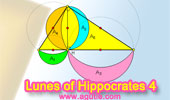Plane Geometry: Pythagoras, Right Triangle, Metric Relations

 Right Triangles Theorems and Problems Index 1Pythagoras and Delaunay Triangulation Art, iPad Apps: Poly. Polygonal Art Illustration. Right Triangles, Formulas and Facts Pythagorean theorem, Similarity, Geometric mean, hypotenuse, cathetus, semiperimeter, altitude, median, similar shapes areas, centers, orthocenter, incenter, centroid, circumcenter, radius, inradius, circumradius, exradius, circles, incircle, circumcircle, excircle. Geometry Problem 1386.Thabit ibn Qurra (826 -901) Theorem and more conclusions, generalized Pythagorean Theorem to any triangle. Geometry Problem 884: The Pythagorean Curiosity Right Triangles and squares, fifteen conclusions. Right triangle, Altitude, Incircle Right Triangle, Altitude to the Hypotenuse, Incircle, Incenter, Inradius, Angle Bisector, Theorems and Problems, Index. Right Triangle Word Cloud. Geometry for Kids. Geometry Problem 1255. Right Triangle, Angle, 90 Degrees, Perpendicular, Collinear Points. Geometry Problem 1253. Triangle, Orthocenter, Circle, Circumcircle, Altitude, Perpendicular, 90 Degree, Concurrency. Geometry Problem 1211 Right Triangle, Altitude, Angle Bisector, 45 Degrees. Geometry Problem 1203 Right Triangle, Square, Angle Bisector, Three Congruent Quadrilaterals. Geometry Problem 1200 Square, Right Triangle, Perpendicular, 90 Degrees, Equal Areas. Geometry Problem 1198 Right Triangle, Square, Rectangle, Quadrilateral, Altitude, Equal Areas. Geometry Problem 1196 Parallelogram, Midpoint, Metric Relations. Geometry Problem 1195 Square, Two Rectangles, Congruence, Metric Relations. Geometry Problem 1194 Equilateral Triangle, Perpendicular, Distance, Side, Metric Relations. Geometry Problem 1165. Right Triangle, Circle, Center, Radius, Cathetus, Perpendicular, 90 Degrees, Perimeter, Metric Relations. Geometry Problem 1159. Triangle, Circumcircle, Sagitta, Chord, Arc, Metric Relations. Geometry Problem 1158. Four Tangent Circles, Tangent Chord, Radius, Metric Relations. Geometry Problem 1157. Four Circles, Mutually Tangent, 90 Degrees, Radius, Metric Relations. Geometry Problem 1156. Square, Tangent Line, 90 Degrees, Metric Relations. Geometry Problem 1155. Right Triangle, Altitude, Incenter, Incircle, Area. Geometry Problem 1154.Sangaku: Three circles and a tangent line. Geometry Problem 1151. Circle, Diameter, Chord, Perpendicular, 90 Degrees, Metric Relations. Dynamic Geometry Problem 1148 Static Geometry Problem 1148. Right Triangle, Incircle, Circumcircle, Tangent, Radius. GeoGebra, HTML5 Animation for Tablets (iPad, Nexus). Geometry Problem 1128. Right Triangles, Medians, Parallel, Angles, Congruence, Half the measure. Geometry Problem 1120. Isosceles Right Triangle, 120 Degree, Angle, Equilateral, Metric Relations. Geometry Problem 1118. Right Triangle, Angle Trisection, Concyclic Points, Cyclic Quadrilateral. Geometry Problem 1117. Right Triangle, Angle Trisection, 90 Degrees, Parallel Lines. Geometry Problem 1116. Right Triangle, Angle Trisection, 90 Degrees, Perpendicular Lines. Geometry Problem 1115. Right Triangle, Angle Trisection, Equilateral Triangle. Geometry Problem 1114. Right Triangle, Angle Trisection, Isosceles, Congruence. Geometry Problem 1111. Right Triangle, External Squares, Cathetus, Angle Bisector, Area, Geometric Mean. The significance of the Pythagorean theorem by Jacob Bronowski. Pythagorean Theorem, 47th Proposition of Euclid's Book I. Mind Map of the Pythagorean Theorem Proofs by shears, translation, similarity. Facts. Go to page: Previous | 1 | 2 | 3 | 4 | 5 | Next

Home | Geometry | Pythagoras Mind Map | Email
Last updated: Sep 22, 2018